Sample application of Nyquist Stability Criteria.

For the examples below, assume that the closed loop system is described by: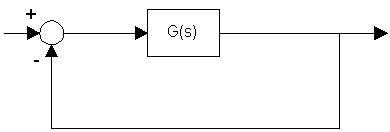Example 1: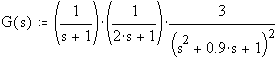the Nyquist plot of G(iw) is shown in Figure 1.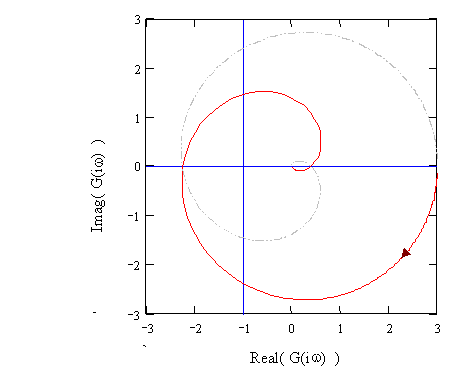Conclusion: The closed loop system: G(s)/(1+G(s) ) is not stable.

To verify the closed loop transfer function is given by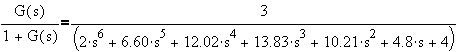where the poles are: -1.293+0.584i , -1.293-0.584i, -0.511+1.303i, -0.511-1.303i, 0.154+0.696i, 0.154-0.696i.

Thus, as predicted, upon closing the loop the system will yield 2 unstable poles.

Example 2: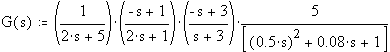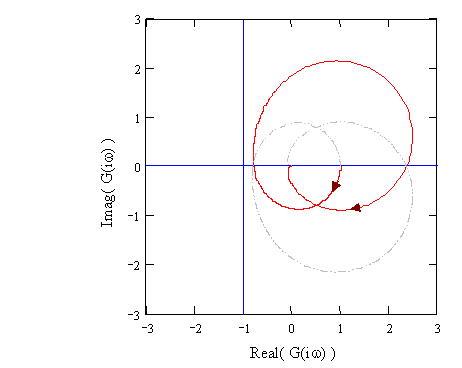Since there are no encirclement of the point -1+0i by G(iw), the system should be stable when the loop is closed.

To verify, the closed loop transfer function is given by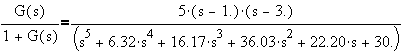where the poles are: -4.318, -0.876+2.269i, -0.876-2.269i, -0.125+1.077i,-0.125-1.077i

Thus, as predicted, upon closing the loop the system should yield no unstable poles.